# A New Favorite Puzzle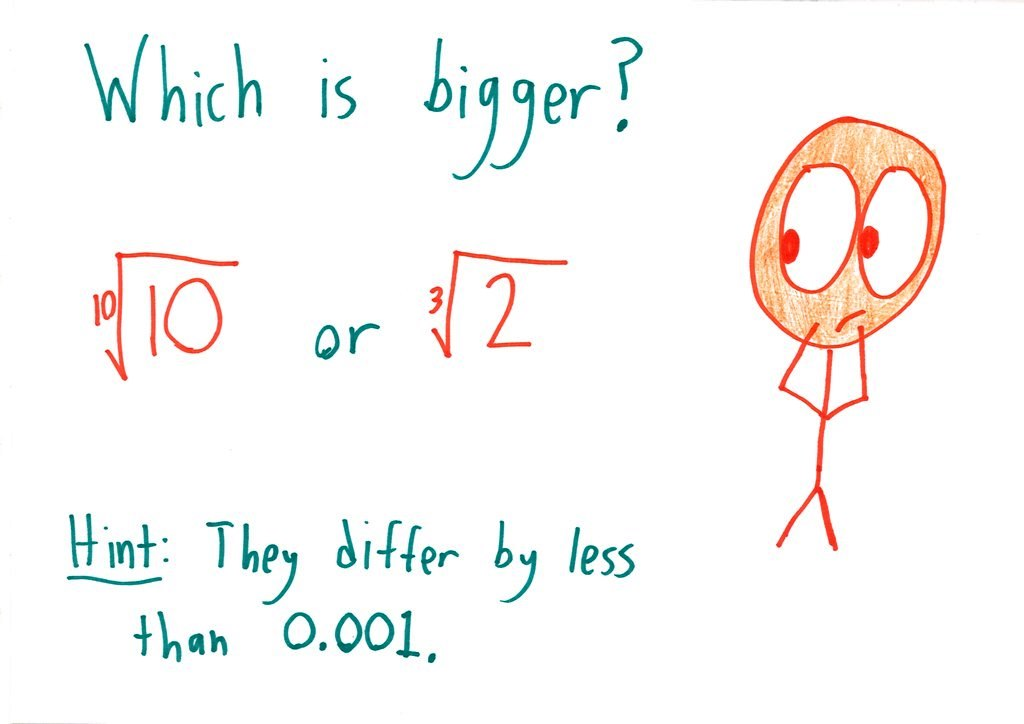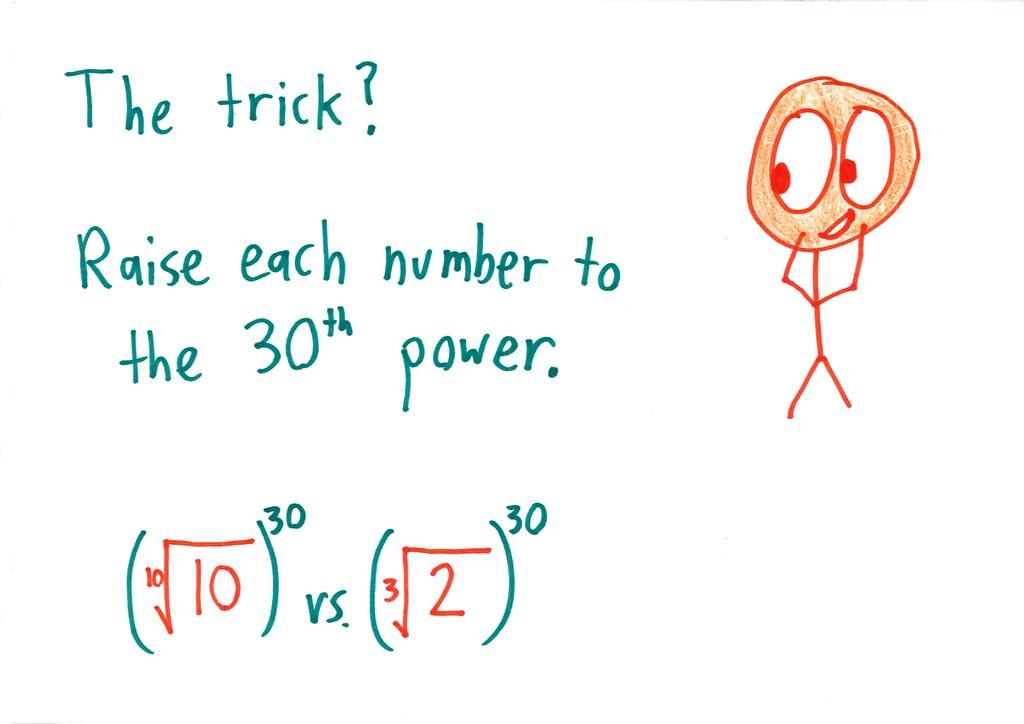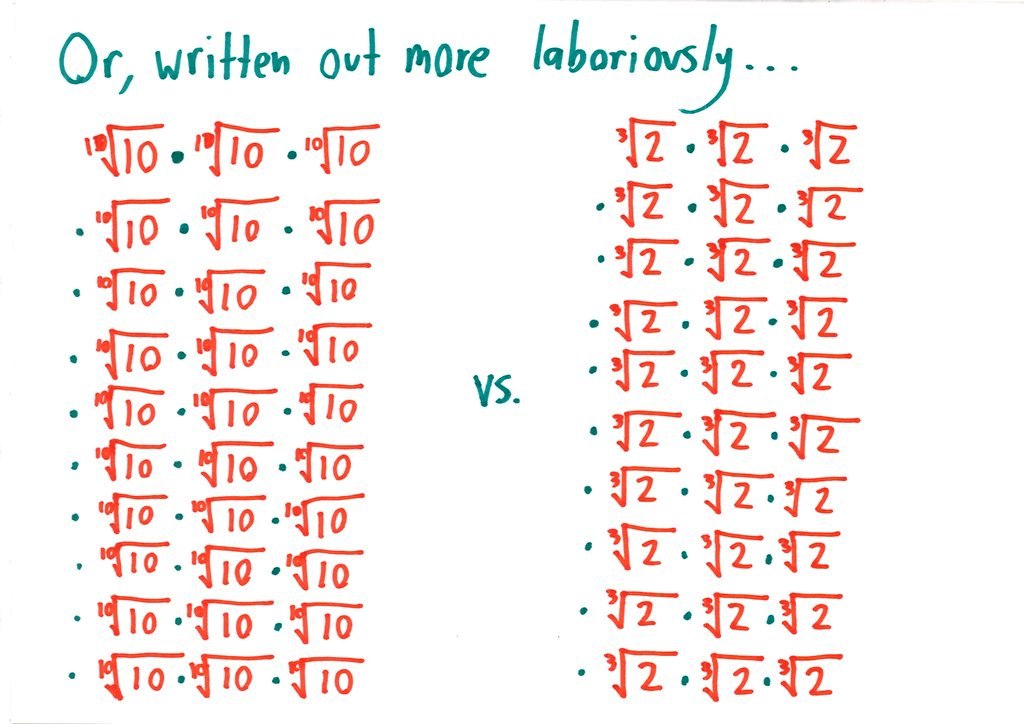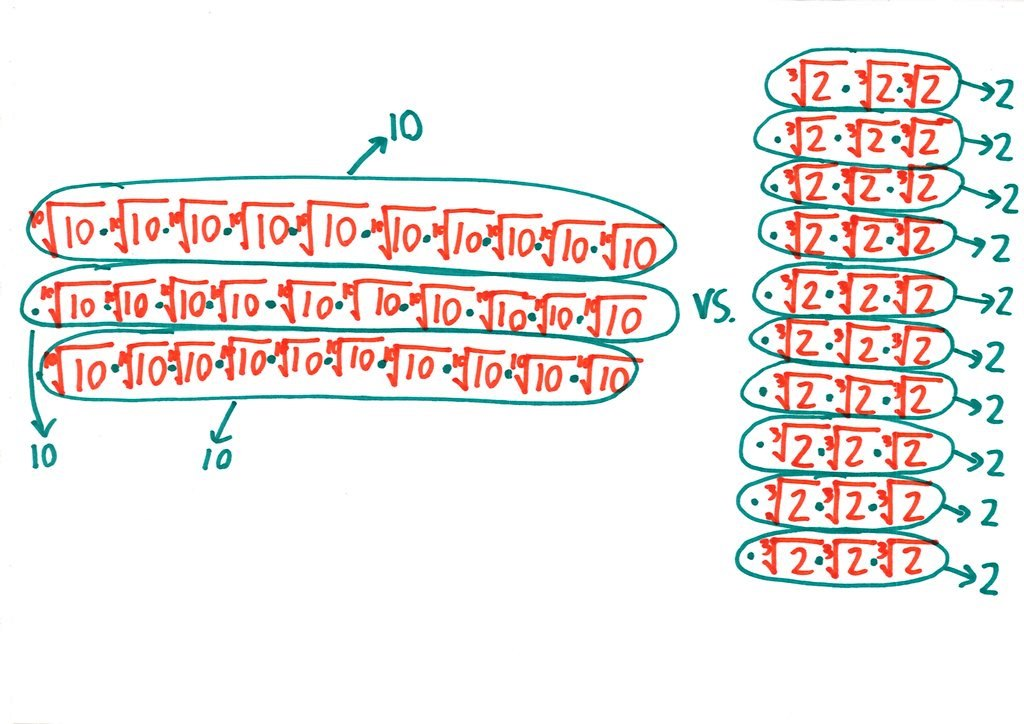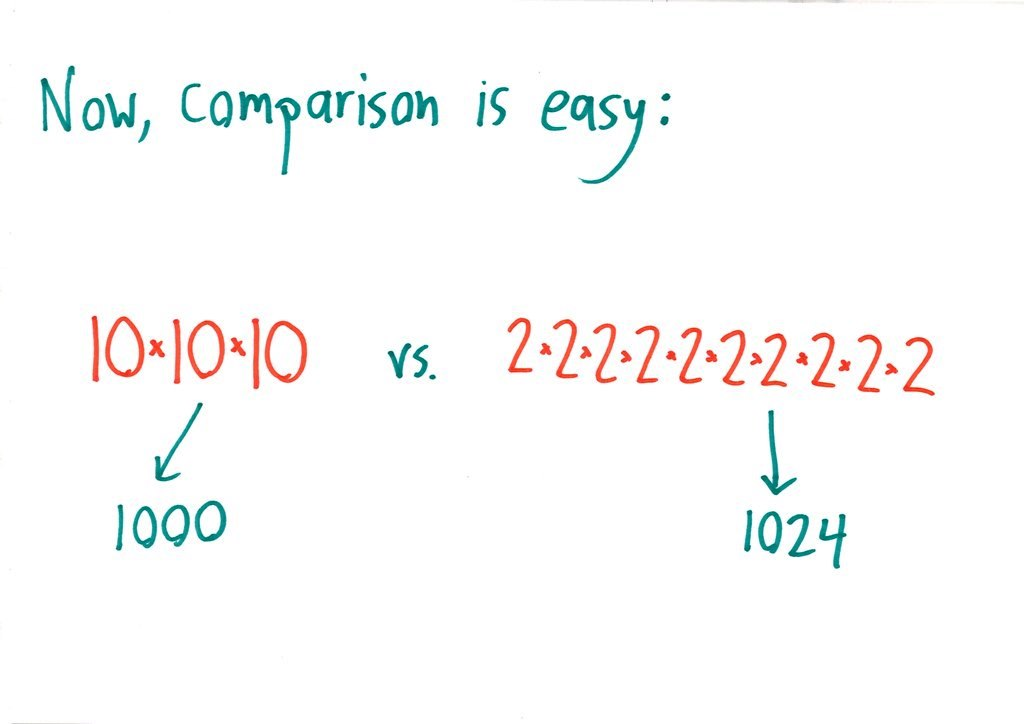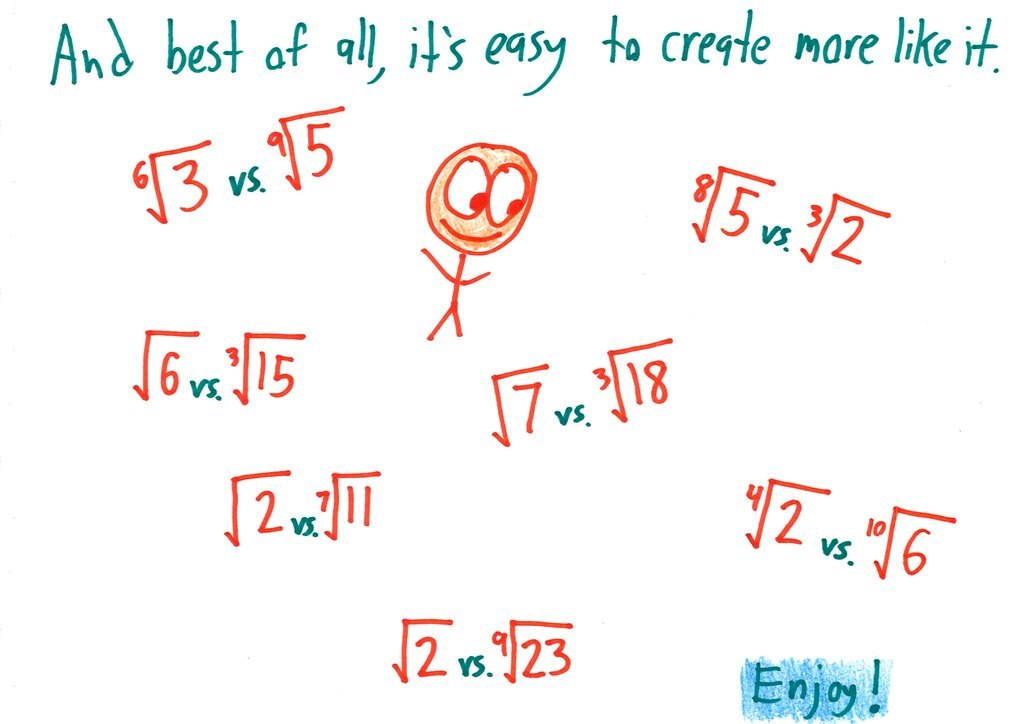## 32 thoughts on “A New Favorite Puzzle”

1.Claire says:

What a nice way to begin my day. I love puzzles! Just be careful when the number under the radical is less than one…

2.howardat58 says:

A noce thing about this is that it mirrors the direct method for comparing two fractions.

1.howardat58 says:

correction: A nice thing …..

3.gblakney says:

Once again, you have made my day! I did not begin to think to “power” my way out of this (or should I say “exponentiate” –which I believe is still legal in the state of North Carolina!)

4.goldenoj says:

We have to know they’re bigger than one, too. So it might be fun to make a reverse puzzle with a fraction!

5.Michael Paul Goldenberg says:

Very nice indeed.

6.BilboBaggins says:

classic maths degree student (or math major for the Yanks out there) I turned straight to logarithms. I much prefer this method though, very nice

1.Chris says:

How did you figure out the logarithm?

7.Pouncer says:

I didn’t notice at first the choice of power (30th) was conveniently chosen to be product of the two powers (10th and 3rd) of the two original terms. In fact the final slide suggested to me that the 30th power should be used, again, to compare fourth root of two and tenth root of six.

Maybe making this “common multiple” thing more explicit would be helpful to others?

8.John Abreu says:

Congratulations for your ´mathwithEXCELLENTdrawing´

9.Ross Presser says:

More quickly: To compare the j’th root of x with the k’th root of y, compare instead x^k with y^j.

10.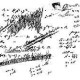Win Smith says:

How about this, with a fraction: which is bigger (3/2)^12 or 2^7?

Bonus question: what does this have to do with J.S. Bach?

1.Doug M says:

I will guess the connection to Bach is that (3/2)^12 is 12 fifths while 2^7 is 7 octaves.

1.Win Smith says:

Yes, if 12 perfect fifths perfectly matched 7 octaves, then consistent musical scales could be constructed with desirable intervals in any key. Because the fifths don’t line up with the octaves, various “temparaments” have been used, including the “well-tempered” approach promoted by Bach.

1.eldermathdragon says:

Ah, I knew it had something to do with octaves and fifths. The factor of two was a dead giveaway. I guess my music theory is a bit non-existent…er, rusty.

2.BilboBaggins says:

My music theory is a bit rusty, remind me why they don’t match up? And what do you mean “consistent musical scales with desirable intervals”?

3.Win Smith says:

Each perfect fifth represents a 3:2 ratio of frequencies. Twelve of these would be a ratio of (3/2)^12 to 1, which is 129.75:1 and not an exact match for 128:1, the frequency ratio for seven octaves (2^7). If the musical math worked out, we could have a keyboard instrument for which every key had perfect fifths, perfect fourths (4:3) and other intervals as ratios of integers. The appeal of these intervals was noted as far back as Pythagoras. A “just” tuning can provide these intervals for one or two keys but other keys will sound terrible.

4.Karen Carlson says:

This video may help with the music/math theory – and it’s fun

11.Doug M says:

My approach was to say that log 2 = 0.301.
1/3 (0.301) which is very nearly 0.1

12.eldermathdragon says:

Great puzzle! It’s remarkable how much of our math, even with irrational numbers, is turning them into integers and comparing them.

13.Jerry Tuttle says:

Nice problem! I think buried within it is the theorem, if x0, then x^z < y^z.

1.Jerry Tuttle says:

Should have said “If x is less than y, and z is greater than zero, then x^z < y^z.," but symbols didn't display properly.

1.Ben Orlin says:

Yeah, I’m with you – the key fact is that raising both sides of an inequality to a power preserves the inequality. (Assuming all numbers are positive.)

Interestingly, a few people have suggested that this property fails if the numbers are between 0 and 1, but in fact this approach handles such a situation without a hitch!

14.Mathemagician says:

5^(1/8) and 2^(1/3) – wouldn’t 5^(1/7) have been more appropriate here?

1.Ben Orlin says:

Yes – good catch! Sloppy error.

15.Meteorit says:

do the “to the power of 30” is still complicated for hand calculation. To the power of 10 is enough and then comparing 10=8*(10/8) and 2^(10/3)=(2^3)*[2^(1/3)]=8*2^1/3; therefore just by comparing 1.25 and 2^1/3

1.RussellColeman says:

Comparing 10^(30/10) to 2^(30/3) isn’t complicated to do by hand. I’d rather compare 10^3 to 2^3 than what you typed.

16.classwithkassandra says:

Intense math in here… Someone bring me. Aboard too

17.tamlknox says:

I solved it a bit differently (less elegantly),, using a bit of algebra. If we say y = 10^(1/10) and x=2^(1/3), then that means x^3 = 2 and y^10 = 10, so if x^10 > 10, we know x is bigger.

Since x^3 = 2, x^10 = x * x^9 = 8x.

For x^10 > 10, then, we just need 8x > 10, or x > 10/8.

So is x bigger or smaller than 10/8?

Well, (10/8)^3 is 1000/512, which is clearly smaller than 2 (which is x^3). So x is indeed bigger than 10/8, and thus x > y.

18.Rene Grothmann says:

Here is another puzzle: Which is bigger pi^e or e^pi. You are not allowed to use a calculator. But: This requires Calculus.

19.Anshul kashyap says:

Nice,I love puzzuls.It is an good method of comprison